# Difference between revisions of "Linear representation theory of general linear group:GL(2,3)"

This article gives specific information, namely, linear representation theory, about a particular group, namely: general linear group:GL(2,3).
View linear representation theory of particular groups | View other specific information about general linear group:GL(2,3)

This article describes the linear representation theory (in characteristic zero and other characteristics excluding 2,3) of general linear group:GL(2,3), which is the general linear group of degree two over field:F3.

## Summary

Item Value
degrees of irreducible representations over a splitting field 1,1,2,2,2,3,3,4
maximum: 4, lcm: 12, number: 8, sum of squares: 48
ring generated by character values (characteristic zero)$\mathbb{Z}[\sqrt{-2}]$, same as$\mathbb{Z}[t]/(t^2 + 2)$
field generated by character values (characteristic zero)$\mathbb{Q}(\sqrt{-2})$, same as$\mathbb{Q}[t]/(t^2 + 2)$

Note that general linear group:GL(2,3) and the binary octahedral group are isoclinic groups of the same order. We know that isoclinic groups have same proportions of degrees of irreducible representations, therefore, in this case, the degrees of irreducible representations are the same for both groups. However, the character tables themselves are not identical. In fact, the fields generated by character values also differ from one another.

## Irreducible representations

### Interpretation as general linear group of degree two

The group is a general linear group of degree two over field:F3. Compare with linear representation theory of general linear group of degree two over a finite field.

Description of collection of representations Parameter for describing each representation How the representation is described Degree of each representation (generic$q$) Degree of each representation ($q = 3$) Number of representations (generic$q$) Number of representations ($q = 3$) Sum of squares of degrees (generic$q$) Sum of squares of degrees ($q = 3$)
One-dimensional, factor through the determinant map a homomorphism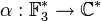$\alpha: \mathbb{F}_3^\ast \to \mathbb{C}^\ast$$x \mapsto \alpha(\det x)$ 1 1$q - 1$ 2$q - 1$ 2
Unclear a homomorphism$\varphi:\mathbb{F}_{9}^\ast \to \mathbb{C}^\ast$ unclear$q - 1$ 2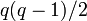$q(q - 1)/2$ 3$q(q - 1)^3/2$ 12
Tensor product of one-dimensional representation and the nontrivial component of permutation representation of$GL_2$ on the projective line over$\mathbb{F}_3$ a homomorphism$\alpha: \mathbb{F}_3^\ast \to \mathbb{C}^\ast$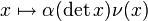$x \mapsto \alpha(\det x)\nu(x)$ where$\nu$ is the nontrivial component of permutation representation of$GL_2$ on the projective line over$\mathbb{F}_3$$q$ 3$q - 1$ 2$q^2(q - 1)$ 18
Induced from one-dimensional representation of Borel subgroup Both distinct representations$\alpha, \beta$ homomorphisms$\mathbb{F}_3^\ast \to \mathbb{C}^\ast$ Induced from the following representation of the Borel subgroup:$\begin{pmatrix} a & b \\ 0 & d \\\end{pmatrix} \mapsto \alpha(a)\beta(d)$$q + 1$ 4$(q - 1)(q - 2)/2$ 1$(q+1)^2(q-1)(q-2)/2$ 16
Total NA NA NA NA$q^2 - 1$ 8$q^4 - q^3 - q^2 + q$ 48

## Character table

FACTS TO CHECK AGAINST (for characters of irreducible linear representations over a splitting field):
Orthogonality relations: Character orthogonality theorem | Column orthogonality theorem
Separation results (basically says rows independent, columns independent): Splitting implies characters form a basis for space of class functions|Character determines representation in characteristic zero
Numerical facts: Characters are cyclotomic integers | Size-degree-weighted characters are algebraic integers
Character value facts: Irreducible character of degree greater than one takes value zero on some conjugacy class| Conjugacy class of more than average size has character value zero for some irreducible character | Zero-or-scalar lemma

In the table below, we denote by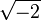$\sqrt{-2}$ a fixed square root of -2.

Representation/conjugacy class representative and size$\begin{pmatrix} 1 & 0 \\ 0 & 1 \\\end{pmatrix}$ (size 1)$\begin{pmatrix} -1 & 0 \\ 0 & -1 \\\end{pmatrix}$ (size 1)$\begin{pmatrix} 0 & 1 \\ -1 & 0 \\\end{pmatrix}$ (size 6)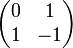$\begin{pmatrix} 0 & 1 \\ 1 & -1 \\\end{pmatrix}$ (size 6)$\begin{pmatrix} 0 & 1 \\ 1 & 1 \\\end{pmatrix}$ (size 6)$\begin{pmatrix} 1 & 1 \\ 0 & 1 \\\end{pmatrix}$ (size 8)$\begin{pmatrix} -1 & 1 \\ 0 & -1 \\\end{pmatrix}$ (size 8)$\begin{pmatrix} 1 & 0 \\ 0 & -1 \\\end{pmatrix}$ (size 12)
trivial 1 1 1 1 1 1 1 1
nontrivial one-dimensional 1 1 1 -1 -1 1 1 -1
two-dimensional (unclear) 2 2 2 0 0 -1 -1 0
two-dimensional (unclear) 2 -2 0$\sqrt{-2}$$-\sqrt{-2}$ -1 1 0
two-dimensional (unclear) 2 -2 0$-\sqrt{-2}$$\sqrt{-2}$ -1 1 0
three-dimensional, factors through standard representation of symmetric group:S4 3 3 -1 -1 -1 0 0 1
three-dimensional, factors through tensor product of standard and sign representations of$S_4$ 3 3 -1 1 1 0 0 -1
four-dimensional, induced from one-dimensional representation of Borel subgroup 4 -4 0 0 0 1 -1 0

## GAP implementation

### Degrees of irreducible representations

The degrees of irreducible representations can be computed using GAP's CharacterDegrees function, as follows:

gap> CharacterDegrees(GL(2,3));
[ [ 1, 2 ], [ 2, 3 ], [ 3, 2 ], [ 4, 1 ] ]

### Character table

The character table can be computed using GAP's CharacterTable function, as follows:

gap> Irr(CharacterTable(GL(2,3)));
[ Character( CharacterTable( GL(2,3) ), [ 1, 1, 1, 1, 1, 1, 1, 1 ] ),
Character( CharacterTable( GL(2,3) ), [ 1, 1, 1, 1, 1, -1, -1, -1 ] ),
Character( CharacterTable( GL(2,3) ), [ 2, -1, 2, -1, 2, 0, 0, 0 ] ),
Character( CharacterTable( GL(2,3) ), [ 2, 1, -2, -1, 0, -E(8)-E(8)^3,
E(8)+E(8)^3, 0 ] ), Character( CharacterTable( GL(2,3) ),
[ 2, 1, -2, -1, 0, E(8)+E(8)^3, -E(8)-E(8)^3, 0 ] ),
Character( CharacterTable( GL(2,3) ), [ 3, 0, 3, 0, -1, 1, 1, -1 ] ),
Character( CharacterTable( GL(2,3) ), [ 3, 0, 3, 0, -1, -1, -1, 1 ] ),
Character( CharacterTable( GL(2,3) ), [ 4, -1, -4, 1, 0, 0, 0, 0 ] ) ]

A visual display of the character table can be achieved as follows:

gap> Display(CharacterTable(GL(2,3)));
CT1

2  4  1  4  1  3  3  3  2
3  1  1  1  1  .  .  .  .

1a 6a 2a 3a 4a 8a 8b 2b

X.1     1  1  1  1  1  1  1  1
X.2     1  1  1  1  1 -1 -1 -1
X.3     2 -1  2 -1  2  .  .  .
X.4     2  1 -2 -1  .  A -A  .
X.5     2  1 -2 -1  . -A  A  .
X.6     3  .  3  . -1  1  1 -1
X.7     3  .  3  . -1 -1 -1  1
X.8     4 -1 -4  1  .  .  .  .

A = -E(8)-E(8)^3
= -Sqrt(-2) = -i2

### Irreducible representations

The irreducible representations of$GL(2,3)$ can be computed using GAP's GAP:IrreducibleRepresentations function, as follows:

gap> IrreducibleRepresentations(GL(2,3));
[ CompositionMapping( [ (4,7)(5,8)(6,9), (2,7,6)(3,4,8) ] -> [ [ [ 1 ] ], [ [ 1 ] ] ], <action isomorphism> ), CompositionMapping( [ (4,7)(5,8)(6,9), (2,7,6)(3,4,8)
] -> [ [ [ -1 ] ], [ [ 1 ] ] ], <action isomorphism> ), CompositionMapping( [ (4,7)(5,8)(6,9), (2,7,6)(3,4,8) ] -> [ [ [ 0, E(3) ], [ E(3)^2, 0 ] ],
[ [ E(3), 0 ], [ 0, E(3)^2 ] ] ], <action isomorphism> ), CompositionMapping( [ (4,7)(5,8)(6,9), (2,7,6)(3,4,8) ] ->
[ [ [ -1/2*E(24)^11-1/2*E(24)^17, -1/2*E(24)-E(24)^11-E(24)^17-1/2*E(24)^19 ], [ -1/2*E(8)-1/2*E(8)^3, 1/2*E(24)^11+1/2*E(24)^17 ] ],
[ [ E(3), E(3) ], [ 0, E(3)^2 ] ] ], <action isomorphism> ), CompositionMapping( [ (4,7)(5,8)(6,9), (2,7,6)(3,4,8) ] ->
[ [ [ E(24)+E(24)^19, -1 ], [ -E(3)+E(3)^2, -E(24)-E(24)^19 ] ], [ [ E(3)+2*E(3)^2, E(8)+E(8)^3 ], [ -E(24)-E(24)^19, -E(3)^2 ] ] ], <action isomorphism> ),
CompositionMapping( [ (4,7)(5,8)(6,9), (2,7,6)(3,4,8) ] -> [ [ [ 0, 0, 1 ], [ 0, -1, 0 ], [ 1, 0, 0 ] ], [ [ 1, 0, 0 ], [ 0, 0, 1 ], [ 1, -1, -1 ] ] ],
<action isomorphism> ), CompositionMapping( [ (4,7)(5,8)(6,9), (2,7,6)(3,4,8) ] -> [ [ [ 0, 1, 0 ], [ 1, 0, 0 ], [ 0, 0, 1 ] ],
[ [ 1, 0, 0 ], [ -1, -1, -1 ], [ 0, 1, 0 ] ] ], <action isomorphism> ), CompositionMapping( [ (4,7)(5,8)(6,9), (2,7,6)(3,4,8) ] ->
[ [ [ 0, 0, -E(3), 0 ], [ 0, 0, -E(3), -E(3) ], [ -E(3)^2, 0, 0, 0 ], [ E(3)^2, -E(3)^2, 0, 0 ] ],
[ [ -E(3)^2, E(3)^2, 0, 0 ], [ -E(3)^2, 0, 0, 0 ], [ 0, 0, E(3)^2, 0 ], [ 0, 0, 1, 1 ] ] ], <action isomorphism> ) ]